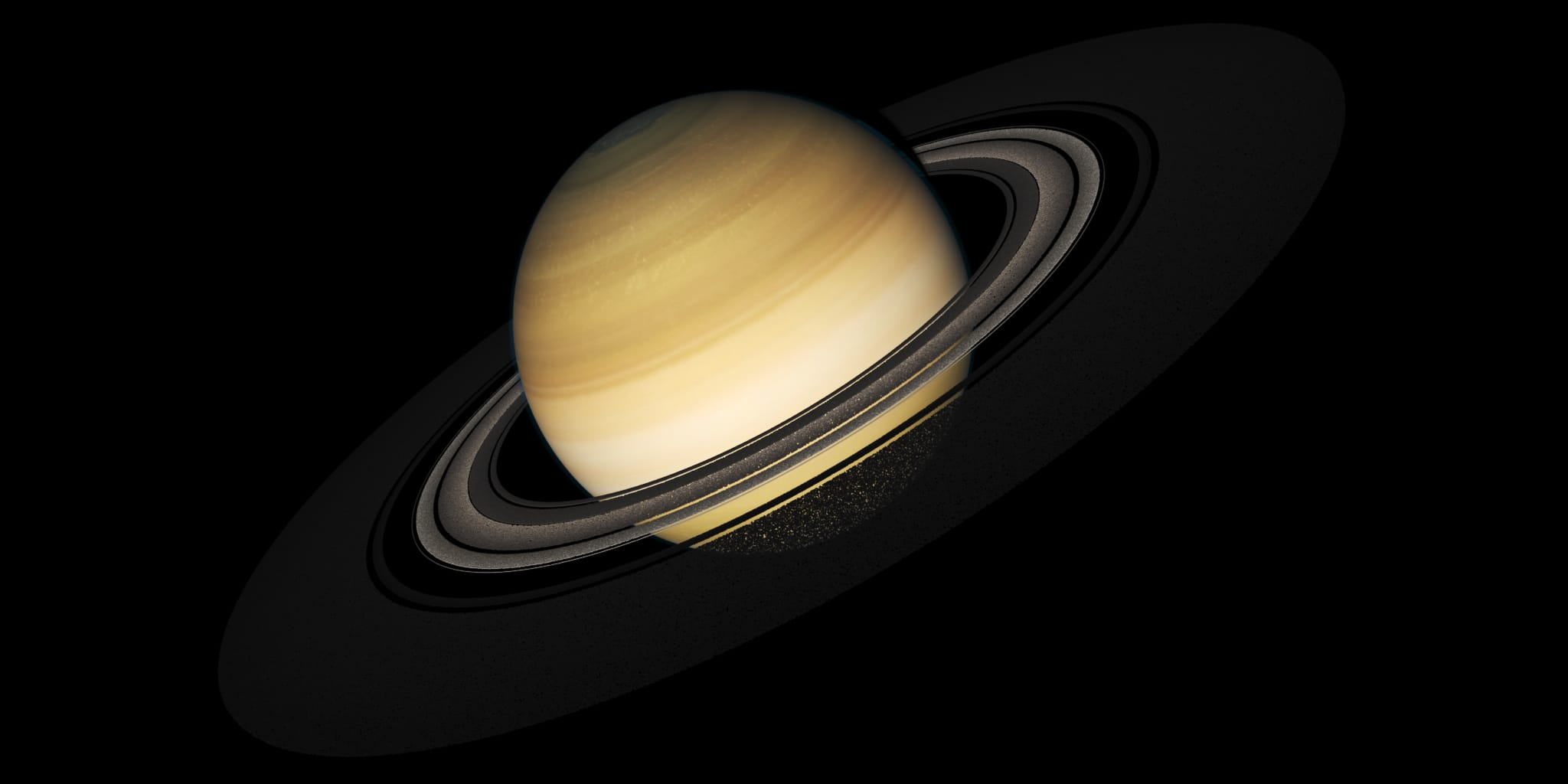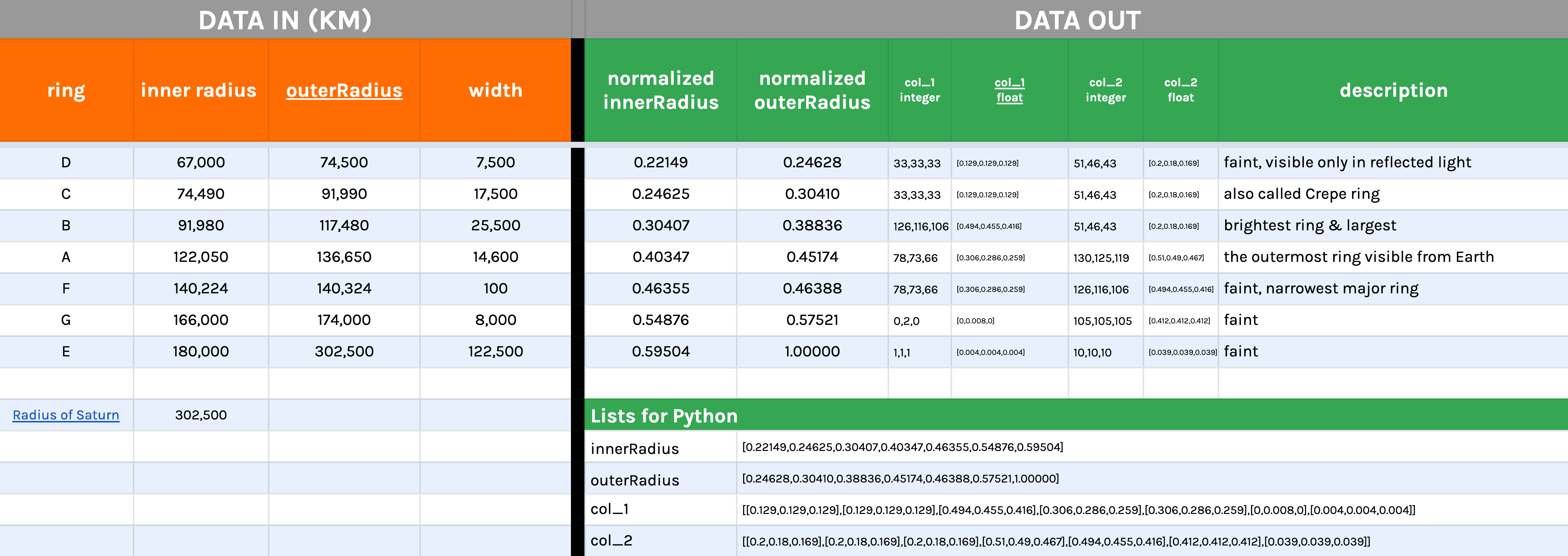## Saturn Rings Point Generation

Generate a rib archive containing point clouds coordinates and color data for Saturn Rings using Python. The rib archive is then rendered in Renderman in Autodesk Maya.

The project began with Professor Malcom Kesson’s writeRing function.

## Researching Saturn Rings DataBefore diving into the code, it was essential to learn more about Saturn and its rings since it has a singular objective form in our world. In my research, I discovered that Saturn has 7 rings with their own respective radii. There are many data available online: NASA, TIVAS, but the one I consulted was

Using Google Sheets and formulas, I normalized the inner and outer radii of each ring by dividing them with the whole planet’s radius. I also added the different colors from the rings into this database. From there, I generate lists/arrays that I can use in my Python functions.

## Generating the rings

The way I wrote my function is to avoid writing multiple writeRing 7 times, I wanted to do that with a for-loop, and have the ability to change the radius without having to change each individual rings’s radii. Hence, that is why I used lists of normalized ratio instead. I also created lists for the start and end colors for each ring.

```				```
# rib_particles.py / writeSaturnRings

def writeSaturnRings(path, s1, s2):
r1 = [0.00, 0.25, 0.30, 0.40, 0.46, 0.55, 0.60]
r2 = [0.25, 0.30, 0.39, 0.45, 0.55, 0.58, 1]
pts = [0.7, 0.7, 1, 1, 1, 1, 3]

# color
col_1 = [[0.129, 0.129, 0.129], [0.129, 0.129, 0.129], [0.494, 0.455, 0.416], [
0.306, 0.286, 0.259], [0.306, 0.286, 0.259], [0.000, 0.008, 0.000], [0.000, 0.008, 0.000]]
col_2 = [[0.200, 0.180, 0.169], [0.200, 0.180, 0.169], [0.200, 0.180, 0.169], [
0.510, 0.490, 0.467], [0.494, 0.455, 0.416], [0.149, 0.149, 0.129], [0.149, 0.149, 0.129]]

numRings = len(r1)

for i in range(numRings):
inner = r1[i] * s2 + s1
outer = r2[i] * s2 + s1
myPts = pts[i] * 20000
newPath = path + '_ring_' + str(i + 1) + '.rib'
writeRing(newPath, myPts, inner, outer, .1, col_1[i], col_2[i])

# write rib that reads all ribs created
```
```

## Rib to read rib archives

Also, just to challenge my comprehension of Python, I wrote a function that generate a rib archive that writes all the directories of the rib archives that was generated from above function. It runs a loop statement based on the number of rings specified. In this case, there are seven of them, and my function appends the rib name with counter variable and a line break.

```				```

rib_file = open(path+'_rings.rib', 'w')
rib_file.write('##bbox: -50.000000 -0.000000 -50.000000 50.000000 0.000000\n\n')
for i in range(num):
rib_file.write('ReadArchive ' + '"' + path + rib_name + str(i+1)+'.rib"\n')
rib_file.close
```
```

### OUTPUT

```				```
##bbox: -50.000000 -0.000000 -50.000000 50.000000 0.000000

```
```

## NORMALIZING DATA WITHIN Python

One mistake that I discovered with the previous method of normalizing the values in Google Sheet is that I do not get the full float values of the data since I rounded them to 5 decimals place. Optimally, I should have prepared the radii data as it is in a CSV format which then can be opened and read in Python. A similar improvement that I made was to write a function that normalizes the values in an array based on the max value input as shown below. This way, I can normalize not just the radii but also the RGB integer values into float (0-1) thus ensuring precision with less human errors of inputing wrong values.

* One thing I also learned about Python regarding float values is that your numbers need have a decimal point in order to calculate float values. For example, 33÷255 will yield 0, but 33.0÷255 will yield 0.129…

```				```
def normalizeValues(arr,maxValue):
#arr must have at least one decimal to print float values
normalized = []
for i in range(len(arr)):
normalized.append(arr[i]/maxValue)
return normalized

normalizeValues([29,200,30],255.0) # RGB float
```
```

### REVISED WRITE FUNCTION

```				```
# rib_particles.py / writeSaturnRings

def writeSaturnRings(path, s1, s2):
r1 = [0.00, 0.25, 0.30, 0.40, 0.46, 0.55, 0.60]
r2 = [0.25, 0.30, 0.39, 0.45, 0.55, 0.58, 1]
pts = [0.7, 0.7, 1, 1, 1, 1, 3]

# color
col_1 = [[0.129, 0.129, 0.129], [0.129, 0.129, 0.129], [0.494, 0.455, 0.416], [
0.306, 0.286, 0.259], [0.306, 0.286, 0.259], [0.000, 0.008, 0.000], [0.000, 0.008, 0.000]]
col_2 = [[0.200, 0.180, 0.169], [0.200, 0.180, 0.169], [0.200, 0.180, 0.169], [
0.510, 0.490, 0.467], [0.494, 0.455, 0.416], [0.149, 0.149, 0.129], [0.149, 0.149, 0.129]]

numRings = len(r1)

for i in range(numRings):
inner = r1[i] * s2 + s1
outer = r2[i] * s2 + s1
myPts = pts[i] * 20000
newPath = path + '_ring_' + str(i + 1) + '.rib'
writeRing(newPath, myPts, inner, outer, .1, col_1[i], col_2[i])

# write rib that reads all ribs created
```
```

## Generating Maya nParticles

Having learn how to write functions and modules to generate rib archive, the next step was to generate particles using the script editor with Maya. The same concept of using the gen_points module still applies, but this time the data could be fed directly into the nParticle coordinates. Below is the new Python module “nParticle.py” that does that and the Python code to input in the Script Editor .

*Ensure that your python scripts are in Maya script folder for the Script Editor to use them.

```				```
// nParticles.py

import gen_points
import math
import maya.cmds as cmds

def writeCubic(num, side):
data = gen_points.cubic(num, side)
cmds.nParticle(p=data)

def writeBox(num, width, height, depth):
data = gen_points.box(num, width, height, depth)
cmds.nParticle(p=data)

cmds.nParticle(p=data)

```
```

### MAYA SCRIPT EDITOR

```				```
import nParticle

nParticle.writeSpherical(200,5)
nParticle.writeCubic(200,4)
nParticle.writeBox(200,10,4,0.2)

```
```

## RENDERS + USING FIELDS

Aside from a Python study, I also got to investigate how to utilize Fields to create interesting design and motion. For the 1st video above, the primary Field that I used was a Radial Field which made all the spheres squished together, and since self-collision was turned on, the form is always in flux. In addition, I added a Vortex and Turbulence Fields to add secondary motion and make it look more organic.

As for the 2nd iteration, I used two spheres as passive colliders and filled the space in between them with nParticles, and used the same set of fields with different settings. I got lots of interesting results from this experiment, below are the most pleasing variants I created.

Finally, to wrap up this whole study, I wrote another function in gen_points.py to generate spiral particles in Maya and implement the nParticles

```				```
// gen_points.py / helix

data = []
n = 0

while n < num:
#theta = n/num
n = float(n+1)
theta = float(n/num*2*math.pi)
x = radius * math.cos(theta*freq)  # math.cos(theta)
z = radius * math.sin(theta*freq)  # math.cos(theta)
y = height * (n/num)  # math.sin(theta)
data.append((x, y, z))
n = n + 1

return data

// nParticle.py